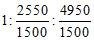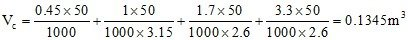# Formulas for calculating quantities of materials

## The formula for calculation of materials for required volume of concrete

Where, Vc = Absolute volume of fully compacted fresh concrete

W =Mass of water

C = Mass of cement

Fa = Mass of fine aggregates

Ca = Mass of coarse aggregates

Sc, Sfa and Sca are the specific gravities of cement, fine aggregates and coarse aggregates respectively.

The air content has been ignored in this calculation.

This method of calculation for quantities of materials for concrete takes into account the mix proportions from design mix or nominal mixes for structural strength and durability requirement.

Now we will learn the material calculation by an example.

Consider concrete with mix proportion of 1:1.5:3 where, 1 is part of cement, 1.5 is part of fine aggregates and 3 is part of coarse aggregates of maximum size of 20mm. The water cement ratio required for mixing of concrete is taken as 0.45.

Assuming bulk densities of materials as follows:

Cement = 1500 kg/m3

Sand = 1700 kg/m3

Coarse aggregates = 1650 kg/m3

## Specific gravities of concrete materials are as follows:

Cement = 3.15

Sand = 2.6

Coarse aggregates = 2.6.

The percentage of entrained air assumed is 2%.

The mix proportion of 1:1.5:3 by dry volume of materials can be expressed in terms of masses as:

Cement = 1 x 1500 = 1500

Sand = 1.5 x 1700 = 2550

Coarse aggregate = 3 x 1650 = 4950.

Therefore, the ratio of masses of these materials w.r.t. cement will as follows == 1 : 1.7 : 3.3

The water cement ratio = 0.45

Now we will calculate the volume of concrete that can be produced with one bag of cement (i.e. 50 kg cement) for the mass proportions of concrete materials.

Thus, the absolute volume of concrete for 50 kg of cement =Thus, for the proportion of mix considered, with on3 bag of cement of 50 kg, 0.1345 m3 of concrete can be produced.

We have considered an entrained air of 2%. Thus the actual volume of concrete for 1 cubic meter of compacted concrete construction will be = 1 -0.02 = 0.98 m3.

Thus, the quantity of cement required for 1 cubic meter of concrete = 0.98/0.1345 = 7.29 bags of cement.

The quantities of materials for 1 m3 of concrete production can be calculated as follows:

The weight of cement required = 7.29 x 50 = 364.5 kg.

Weight of fine aggregate (sand) = 1.5 x 364.5 = 546.75 kg.

Weight of coarse aggregate = 3 x 364.5 = 1093.5 kg.

### 1 Comment

1.Mebratu Abate says:

I aprshet you

This site uses Akismet to reduce spam. Learn how your comment data is processed.